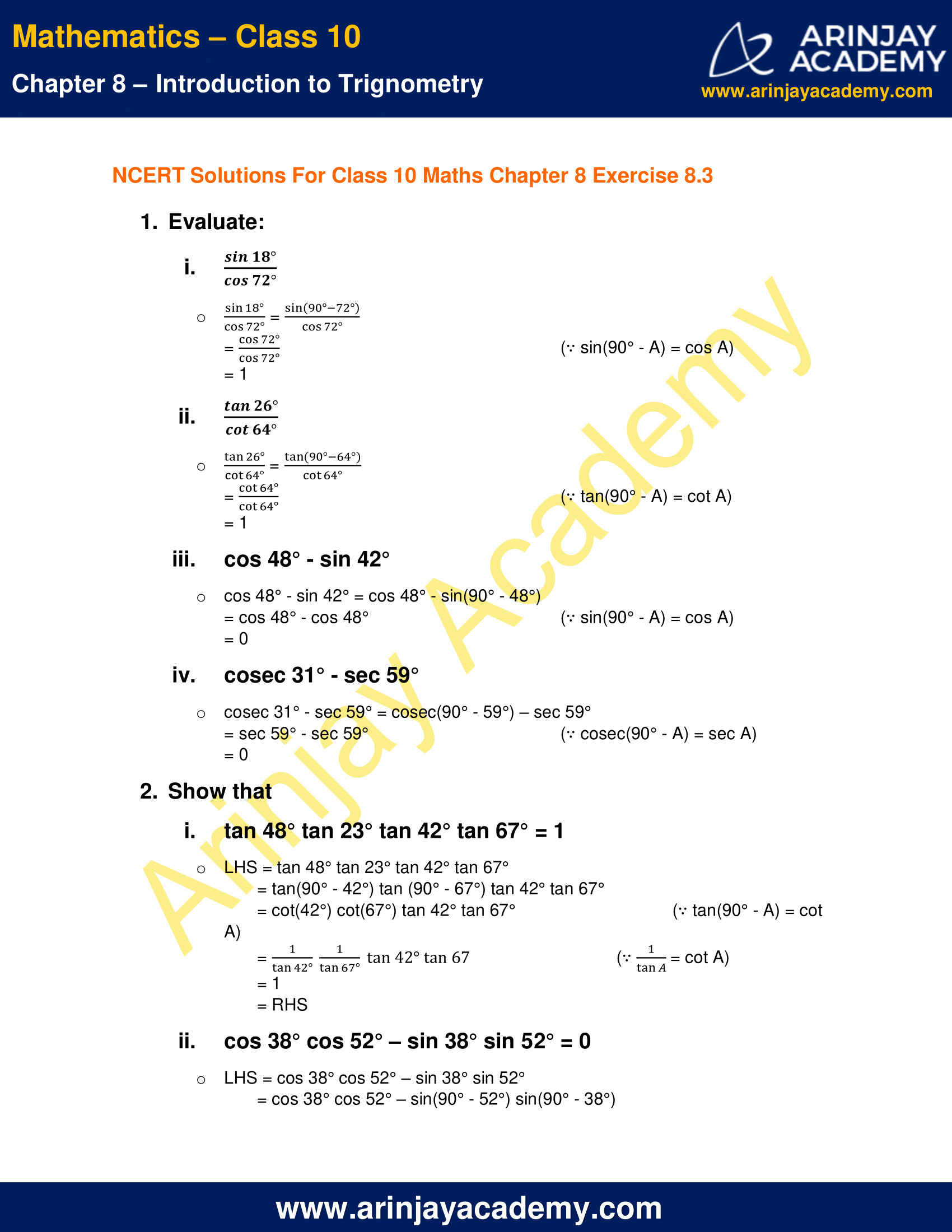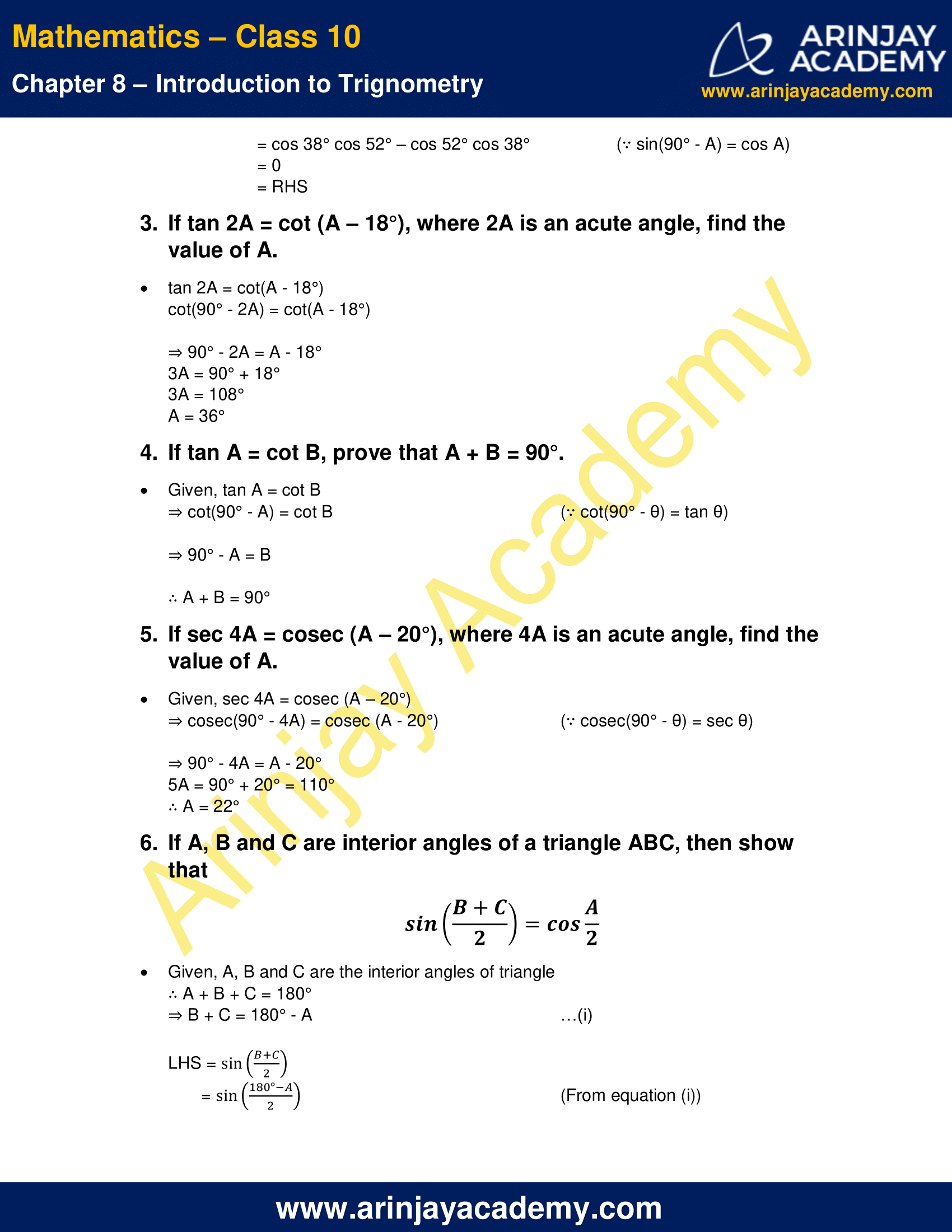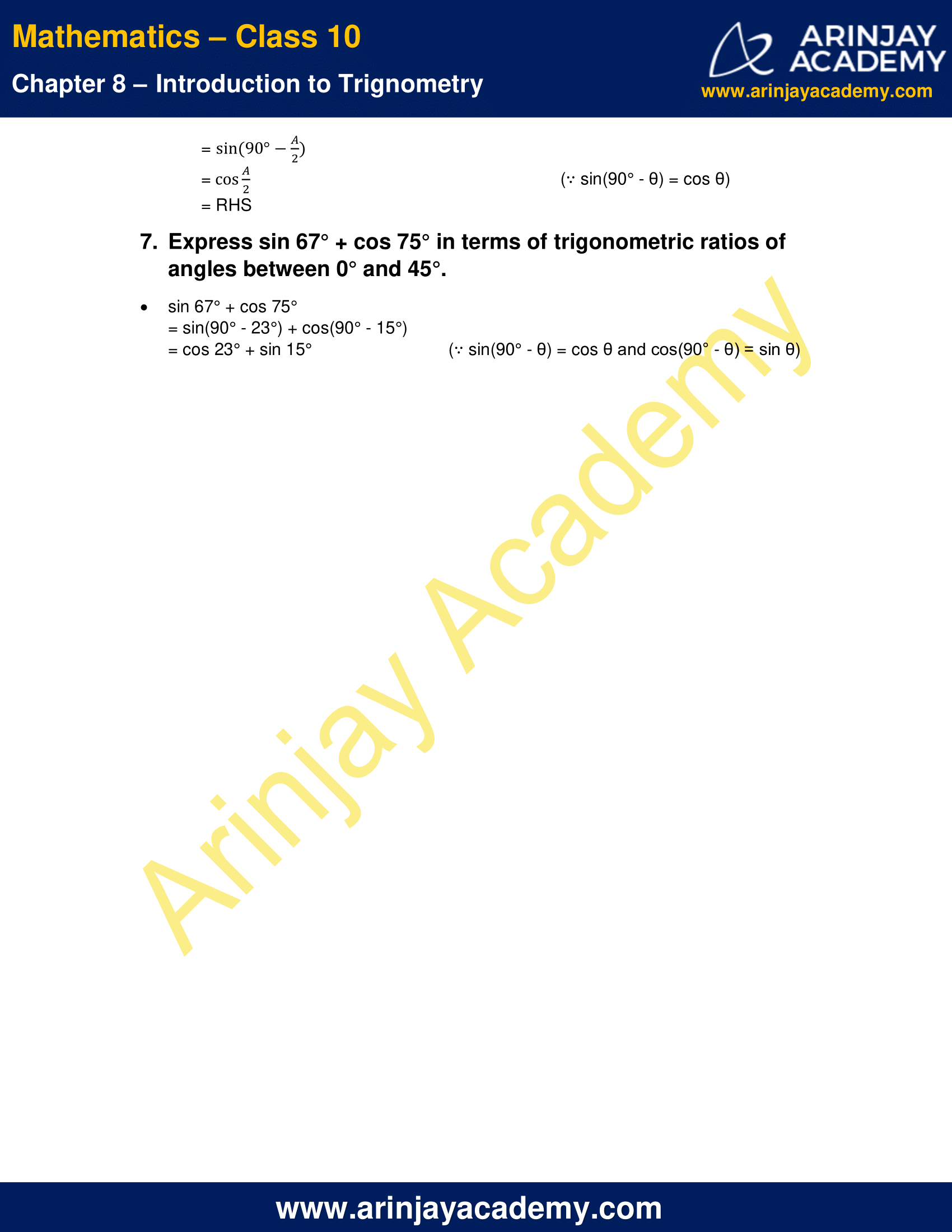# NCERT Solutions For Class 10 Maths Chapter 8 Exercise 8.3 – Introduction to Trignometry

Download NCERT Solutions For Class 10 Maths Chapter 8 Exercise 8.3 – Introduction to Trignometry. This Exercise contains 7 questions, for which detailed answers have been provided in this note. In case you are looking at studying the remaining Exercise for Class 10 for Maths NCERT solutions for other Chapters, you can click the link at the end of this Note.

### NCERT Solutions For Class 10 Maths Chapter 8 Exercise 8.3 – Introduction to TrignometryNCERT Solutions For Class 10 Maths Chapter 8 Exercise 8.3 – Introduction to Trignometry

1. Evaluate:

i.$\cfrac { sin{ 18 }^{ \circ } }{ cos72^{ \circ } }$
=$\cfrac { sin({ 90 }^{ \circ }-72^{ \circ }) }{ cos72^{ \circ } }$
=$\cfrac { cos72^{ \circ } }{ cos72^{ \circ } }$  (∵ sin(90° – A) = cos A)
= 1

ii.$\cfrac { tan{ 26 }^{ \circ } }{ cot64^{ \circ } }$
=$\cfrac { tan{ 26 }^{ \circ } }{ cot64^{ \circ } }$
=$\cfrac { tan({ 90 }^{ \circ }-64^{ \circ }) }{ cot64^{ \circ } }$
=$\cfrac { cot64^{ \circ } }{ cot64^{ \circ } }$  (∵ tan(90° – A) = cot A)
= 1

iii. cos 48° – sin 42°

cos 48° – sin 42° = cos 48° – sin(90° – 48°)
= cos 48° – cos 48°    (∵ sin(90° – A) = cos A)
= 0

iv. cosec 31° – sec 59°

cosec 31° – sec 59° = cosec(90° – 59°) – sec 59°
= sec 59° – sec 59°   (∵ cosec(90° – A) = sec A)
= 0

2. Show that

i. tan 48° tan 23° tan 42° tan 67° = 1

LHS = tan 48° tan 23° tan 42° tan 67°
= tan(90° – 42°) tan (90° – 67°) tan 42° tan 67°
= cot(42°) cot(67°) tan 42° tan 67°    (∵ tan(90° – A) = cot A)$\cfrac { 1 }{ tan42^{ \circ } } \cfrac { 1 }{ tan67^{ \circ } }$tan42°tan67°       (∵$\cfrac { 1 }{ tanA^{ \circ } }$= cot A)
= 1
= RHS

ii. cos 38° cos 52° – sin 38° sin 52° = 0
LHS = cos 38° cos 52° – sin 38° sin 52°
= cos 38° cos 52° – sin(90° – 52°) sin(90° – 38°)
= cos 38° cos 52° – cos 52° cos 38°  (∵ sin(90° – A) = cos A)
= 0
= RHS

3. If tan 2A = cot (A – 18°), where 2A is an acute angle, find the value of A.

tan 2A = cot(A – 18°)
cot(90° – 2A) = cot(A – 18°)
⇒ 90° – 2A = A – 18°
3A = 90° + 18°
3A = 108°
A = 36°

4. If tan A = cot B, prove that A + B = 90°.

Given, tan A = cot B
⇒ cot(90° – A) = cot B     (∵ cot(90° – θ) = tan θ)
⇒ 90° – A = B
∴ A + B = 90°

5. If sec 4A = cosec (A – 20°), where 4A is an acute angle, find the value of A.

Given, sec 4A = cosec (A – 20°)
⇒ cosec(90° – 4A) = cosec (A – 20°)    (∵ cosec(90° – θ) = sec θ)
⇒ 90° – 4A = A – 20°
5A = 90° + 20° = 110°
∴ A = 22°

6. If A, B and C are interior angles of a triangle ABC, then show that$sin\left( \cfrac { B+C }{ 2 } \right)$ =$cos\left( \cfrac { A }{ 2 } \right)$

Given, A, B and C are the interior angles of triangle
∴ A + B + C = 180°
⇒ B + C = 180° – A   …(i)

LHS =$sin\left( \cfrac { B+C }{ 2 } \right)$
=$sin\left( \cfrac { { 180 }^{ \circ }-A }{ 2 } \right)$ (From equation (i))
=$sin\left( 90^{ \circ }-\cfrac { A }{ 2 } \right)$$cos\cfrac { A }{ 2 }$ (∵ sin(90° – θ) = cos θ)
= R.H.S

7. Express sin 67° + cos 75° in terms of trigonometric ratios of angles between 0° and 45°.

sin 67° + cos 75°
= sin(90° – 23°) + cos(90° – 15°)
= cos 23° + sin 15°   (∵ sin(90° – θ) = cos θ and cos(90° – θ) = sin θ)

NCERT Solutions for Class 10 Maths Chapter 8 Exercise 8.3 – Introduction to Trignometry, has been designed by the NCERT to test the knowledge of the student on the topic – Trigonometric Ratios of Complementary Angles

Download NCERT Solutions For Class 10 Maths Chapter 8 Exercise 8.3 – Introduction to Trignometry